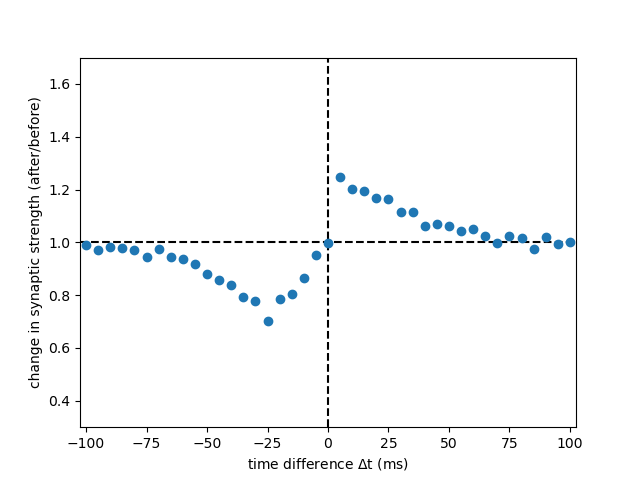# Example: Graupner_Brunel_2012

Note

You can launch an interactive, editable version of this example without installing any local files using the Binder service (although note that at some times this may be slow or fail to open):Fig. 2 C, panel DP from:

Calcium-based plasticity model explains sensitivity of synaptic changes to spike pattern, rate, and dendritic location

PNAS 109 (10): 3991-3996 (2012) https://doi.org/10.1073/pnas.1109359109

by Graupner M. and Brunel N. (2012)

For the noise term see corrections https://www.pnas.org/doi/10.1073/pnas.1220044110.

For the original implementations see https://github.com/mgraupe/CalciumBasedPlasticityModel/tree/main/Graupner2012PNAS.

Sebastian Schmitt, 2022

import multiprocessing

import numpy as np
import matplotlib.pyplot as plt

from brian2 import NeuronGroup, Synapses
from brian2 import ms, second
from brian2 import run

# number of time differences in STDP plot
POINTS = 41

# maximal time difference
STDP_DT_MAX = 100 * ms

# (symmetric) minimal time difference
STDP_DT_MIN = -STDP_DT_MAX

# number of repetitions
REPETITIONS = 1000

# time difference step size
STDP_DT_STEP = (STDP_DT_MAX - STDP_DT_MIN) / (POINTS - 1)

def run_sim(point_index):
"""Run simulation for one STDP time difference"""

# set up two groups of neurons, G spikes at fixed times starting from STDP_DT_MAX
# H spikes shifted according to point_index and has as many neurons as REPETITIONS*2
# (we need to multiply by 2 for both initial states (UP and DOWN))
# G:    |
# H: |
# H: |
# H: |
# ...
G = NeuronGroup(1, "", threshold=f"t>{STDP_DT_MAX/ms}*ms", refractory=1 * second)
H = NeuronGroup(
REPETITIONS * 2, "tspike:second", threshold="t>tspike", refractory=1 * second
)
H.tspike = [point_index * STDP_DT_STEP] * REPETITIONS * 2

synapses_eqs = """
tau      : second (constant, shared)
rho_star : 1      (constant, shared)
gamma_p  : 1      (constant, shared)
theta_p  : 1      (constant, shared)
gamma_d  : 1      (constant, shared)
theta_d  : 1      (constant, shared)
drho/dt = (-rho*(1-rho)*(rho_star-rho)
+ gamma_p*(1-rho)*int((c - theta_p) > 0)
- gamma_d*rho*int((c-theta_d) > 0)
+ sigma*sqrt(tau)*sqrt(int((c-theta_d) > 0) + int((c-theta_p) > 0))*xi
) / tau : 1 (clock-driven)
dc/dt = -c/tau_Ca  : 1 (clock-driven)
tau_Ca : second (constant, shared)
sigma  : 1      (constant, shared)
"""

C_pre = 1
C_post = 2
D = 13.7 * ms

synapses = Synapses(
G,
H,
model=synapses_eqs,
on_pre="c += C_pre",
on_post="c += C_post",
delay=D,
method="heun",
)

synapses.connect()

synapses.tau_Ca = 20 * ms
synapses.theta_d = 1
synapses.theta_p = 1.3
synapses.gamma_d = 200
synapses.gamma_p = 321.808
synapses.sigma = 2.8284
synapses.tau = 150 * second
synapses.rho_star = 0.5

# start with equal number of synapses in DOWN and UP state
# must match b in analysis below
rho_initial = np.array( * REPETITIONS +  * REPETITIONS)
synapses.rho = rho_initial

def report_callback(elapsed, completed, start, duration):
print(
f"time difference {(point_index*STDP_DT_STEP - STDP_DT_MAX)/ms:.0f} ms is {completed:2.0%} done"
)

run(60 * second, report=report_callback)

return synapses.rho[:], rho_initial

if __name__ == "__main__":

with multiprocessing.Pool() as p:
results = p.map(run_sim, range(POINTS))

# initial fraction of synapses in DOWN state
beta = 0.5

# ratio of UP and DOWN state weights (w1/w0)
b = 5

change_in_syn_strengths = []

for rhos, rhos_initial in results:

# average switching probabilities
U = np.mean(rhos[rhos_initial < 0.5] > 0.5)
D = np.mean(rhos[rhos_initial > 0.5] < 0.5)

change_in_syn_strength = (
(1 - U) * beta + D * (1 - beta) + b * (U * beta + (1 - D) * (1 - beta))
) / (beta + (1 - beta) * b)

change_in_syn_strengths.append(change_in_syn_strength)

stdp_dts = [
point_index * STDP_DT_STEP - STDP_DT_MAX for point_index in range(POINTS)
]

plt.axvline(0, linestyle="dashed", color="k")
plt.axhline(1, linestyle="dashed", color="k")
plt.plot(stdp_dts / ms, change_in_syn_strengths, marker="o", linestyle="None")
plt.xlim(
(STDP_DT_MIN - STDP_DT_STEP / 2) / ms, (STDP_DT_MAX + STDP_DT_STEP / 2) / ms
)
plt.ylim(0.3, 1.7)
plt.xlabel(r"time difference $\Delta$t (ms)")
plt.ylabel("change in synaptic strength (after/before)")
plt.show()